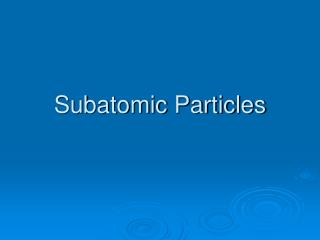DownloadDownload PresentationSubatomic Particles

# Subatomic Particles

Télécharger la présentation## Subatomic Particles

- - - - - - - - - - - - - - - - - - - - - - - - - - - E N D - - - - - - - - - - - - - - - - - - - - - - - - - - -
##### Presentation Transcript

1. Subatomic Particles

2. Subatomic Particles • subatomic-lower (or smaller) than an atom • Protons-positive particles • Neutrons-neutral particles • Electrons-negative particles

3. Location of particles • Protons and neutrons are in the nucleus (core) of the atom. • electrons are buzzing around the nucleus in the electron cloud or shell. • The nucleus makes up 99.99% of the mass of the atom. • You compared to pocket lint • The nucleus is 1/100,000 of the volume of an atom • a marble compared to a football stadium

4. Mass of particles • Since subatomic particles are so small they cannot be measured in grams • instead they are measured in atomic mass units or amu • 1 amu = 1.61x10-24 g • remember 1 g is about the mass of a paper clip

5. Table of particles

6. Determining the number of subatomic particles in an atom

7. Determining the number of protons • Atomic number (the number the periodic table is arranged by) is the number of protons in an atom. • This number cannot change for a given element without changing the element. • Oxygen will always have 8 p+, He will always have 2 p+.

8. Determining electrons • If the atom is neutral, the number of protons equal the number of electrons. • Therefore copper has 29 e- • Krypton has 36 e- • Electrons are the easiest to add or remove from an atom. • This number can be different from atom to atom

9. Ions • Charged Particles (atoms that are not neutral) • They can be made by changing the number of electrons NOT protons!! • Sr2+ or Sr++ means strontium with a 2+ charge on it. • Strontium with 38 protons and 36 electrons • O2- or O -- • Oxygen with 8 protons and 10 electrons

10. Determining the number of neutrons in an atom • The atomic mass number is the number of protons + the number of neutrons. • mass number – atomic number = # of no • Aluminum has a mass number of 27 and an atomic number of 13, how many neutrons? • 14 • The number of neutrons is slightly variable in a given element.

11. Number of particles in an atom • carbon has a mass number of 12 and an atomic number of 6, how many neutrons, protons and electrons? • no =6, e- = 6, p+ = 6 • Lithium has a mass number of 7 and an atomic number of 3 how many neutrons, protons and electrons? • no =4, e- = 3, p+ = 3

12. Number of particles in an atom • Chlorine has a mass number of 35 and an atomic number of 17, how many neutrons, protons and electrons? • no =18, e- = 17, p+ = 17 • Neon has a mass number of 20 and an atomic number of 10 how many neutrons, protons and electrons? • no =10, e- = 10, p+ = 10

13. Number of particles in an ion • Ion- charged particle (atom with a different number of electrons) • Cl- mass number- 35 atomic number- 17 • no =18, e- = 18, p+ = 17 • Be2+mass number- 9 atomic number- 4 • no =5, e- = 2, p+ = 4 • B3+ mass number- 11 atomic number- 5 • no =6, e- = 2, p+ = 5

14. Back to neutrons being slightly variable • Isotope • ~Atoms of the same element with a different number of neutrons. • If you grabbed 100 Mg atoms you would find 30 had 13 no and 70 had 12 no. • 70% of the atoms have a mass of 24 amu, 30% have a mass of 25 amu.

15. Decimal Mass numbers • On the periodic table, mass numbers are decimals. • They are averages. • Mg • 70 x 24 = 1680 • 30 x 25 = 750 • 2430 =24.3 100

16. Another example • Carbon • out of 200 carbon atoms… • 199 would have a mass of 12 • 1 would have a mass of 14 • so the mass number would be… 12x 199 = 2388 ____ 14 x 1 = 14 2402 /200 = 12.01 amu

17. Quick Review • protons- atomic number =# of p+, this is the only number that cannot change for an element. • electrons- if the atom is neutral then # of p+= # of e-. If it has a charge change this number to agree. • neutrons- mass number – atomic number = # of no • Do not use the mass number from the periodic table.